# Exam III Physics 101 Lecture 21 Waves Todays

• Slides: 21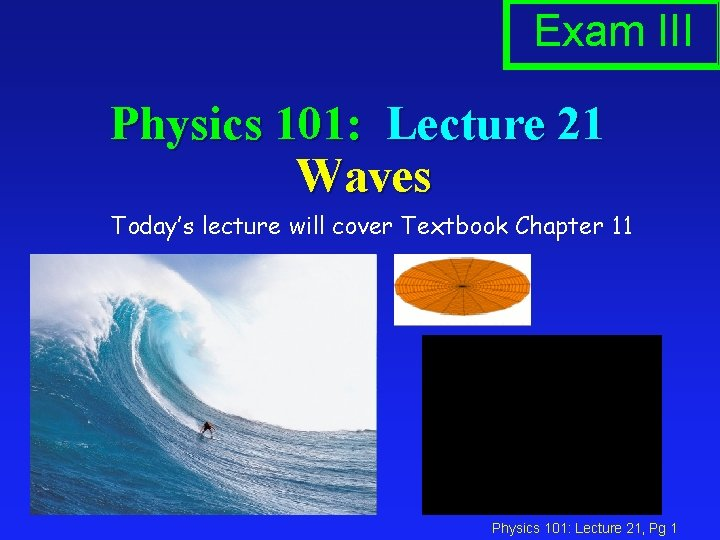Exam III Physics 101: Lecture 21 Waves Today’s lecture will cover Textbook Chapter 11 Physics 101: Lecture 21, Pg 1HE 2 Results Physics 101: Lecture 21, Pg 2Projection based on a previous semester Avg (ex 1&2), max grade, median grade, min grade 53 - 56 C-, D-, F N: 10 57 - 60 C, D+, F N: 18 61 - 64 C+, D+, F N: 19 65 - 68 B+, C, D N: 37 69 - 72 A-, C, D- N: 52 73 - 76 A-, C+, D- N: 52 77 - 80 A, B, D- N: 55 81 - 84 A+, B+, C- N: 39 85 - 88 A+, A-, D- N: 47 89 - 92 A+, A, C N: 32 93 - 96 A+, A- N: 22 97 -100 A+, A+ N: 03 Physics 101: Lecture 21, Pg 3Hour Exam 3 April 25. Covers Lectures 17 -24 (8 Lectures) Finals will cover everything including the new materials in Lectures 25 -28. Physics 101: Lecture 21, Pg 4Preflight 5 Which concepts did you find the most difficult when preparing for this lecture? there was a lot of info Standing waves was difficult to understand. What does it mean and can you give another explanation as to how to calculate it? Also, reflection was not explained enough. What is the difference between a fixed and a free boundary? Overtones! harmonic waves. Longitudinal waves. Everything… Not paying attention Physics 101: Lecture 21, Pg 5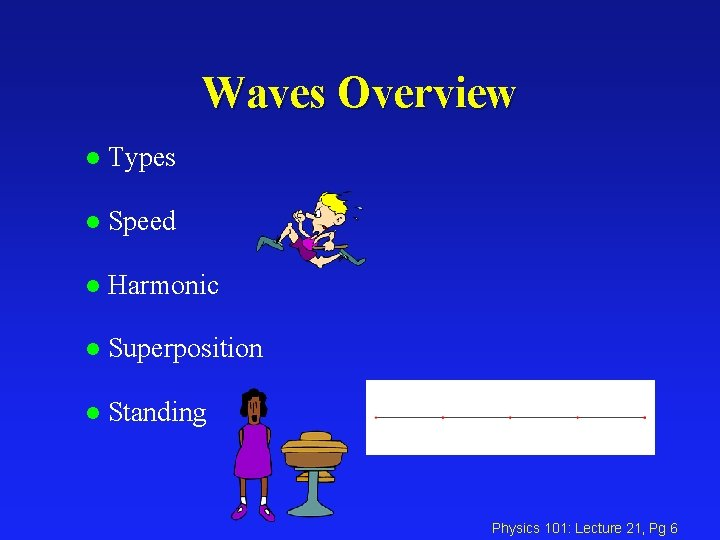Waves Overview l Types l Speed l Harmonic l Superposition l Standing Physics 101: Lecture 21, Pg 6Types of Waves l Transverse: The medium oscillates perpendicular to the direction the wave is moving. èWater (more or less) èSlinky demo l Longitudinal: The medium oscillates in the same direction as the wave is moving èSound èSlinky demo Physics 101: Lecture 21, Pg 7 8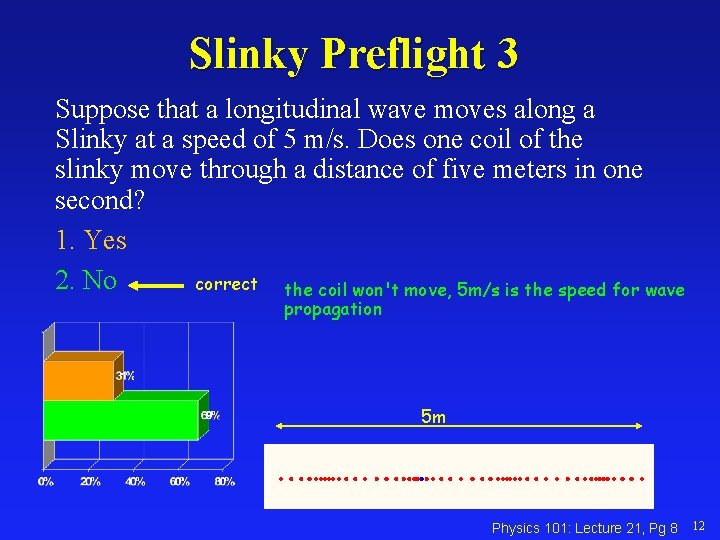Slinky Preflight 3 Suppose that a longitudinal wave moves along a Slinky at a speed of 5 m/s. Does one coil of the slinky move through a distance of five meters in one second? 1. Yes 2. No correct the coil won't move, 5 m/s is the speed for wave propagation 5 m Physics 101: Lecture 21, Pg 8 12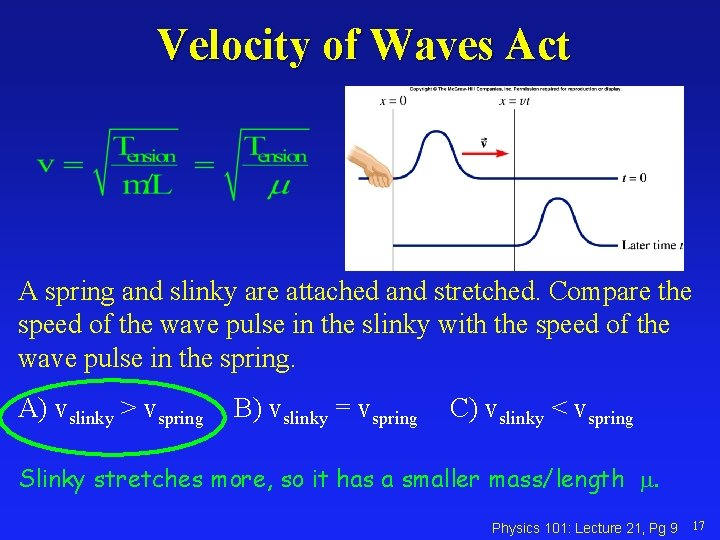Velocity of Waves Act A spring and slinky are attached and stretched. Compare the speed of the wave pulse in the slinky with the speed of the wave pulse in the spring. A) vslinky > vspring B) vslinky = vspring C) vslinky < vspring Slinky stretches more, so it has a smaller mass/length m. Physics 101: Lecture 21, Pg 9 17Harmonic“the. Waves weird/new symbols get me y(x, t) = A cos(wt –kx) confused i never know what they represent “ Wavelength: The distance between identical points on the wave. Amplitude: The maximum displacement A of a point on the wave. Angular Frequency w: w = 2 p f Wave Number k: k = 2 p / Recall: f = v / y Wavelength Amplitude A A x Physics 101: Lecture 21, Pg 10 20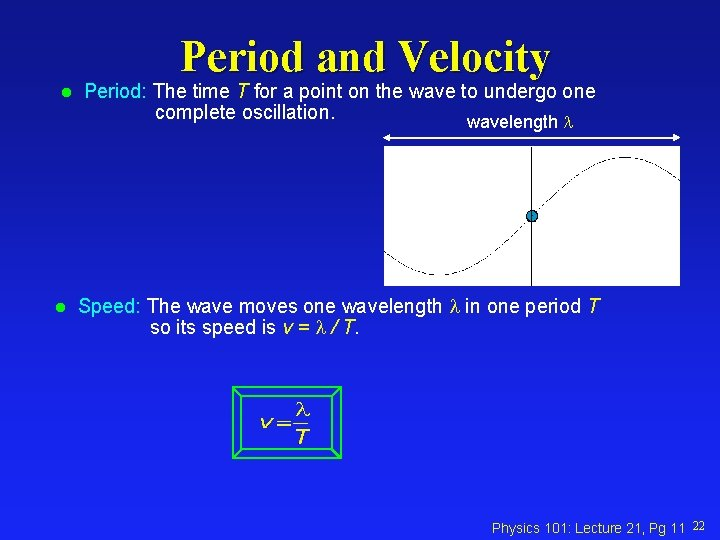Period and Velocity l l Period: The time T for a point on the wave to undergo one complete oscillation. wavelength Speed: The wave moves one wavelength in one period T so its speed is v = / T. Physics 101: Lecture 21, Pg 11 22Harmonic Waves Exercise y(x, t) = A cos(wt –kx) Label axis and tic marks if the graph shows a snapshot of the wave y(x, t) = 2 cos(4 t – 2 x) at x=0. T = 2 p / w Recall: T = 2 p /w = 2 p/ 4 = 1. 58 +2 p/4 -2 p/2 3 p/4 t Physics 101: Lecture 21, Pg 12 25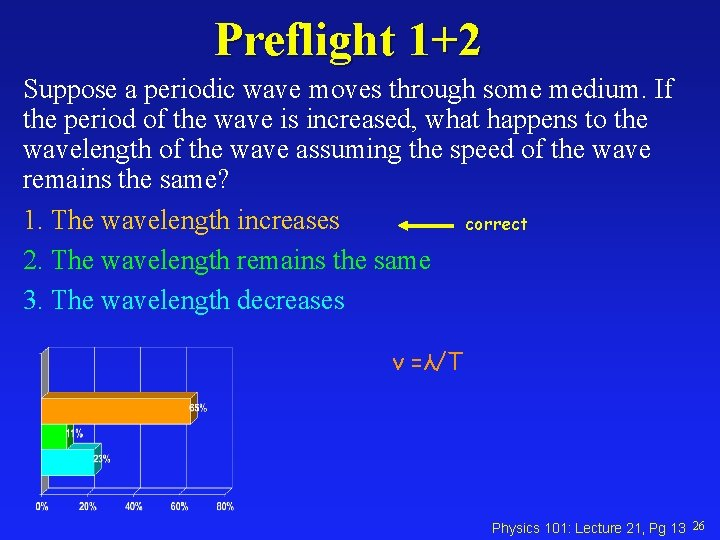Preflight 1+2 Suppose a periodic wave moves through some medium. If the period of the wave is increased, what happens to the wavelength of the wave assuming the speed of the wave remains the same? 1. The wavelength increases correct 2. The wavelength remains the same 3. The wavelength decreases v =λ/T Physics 101: Lecture 21, Pg 13 26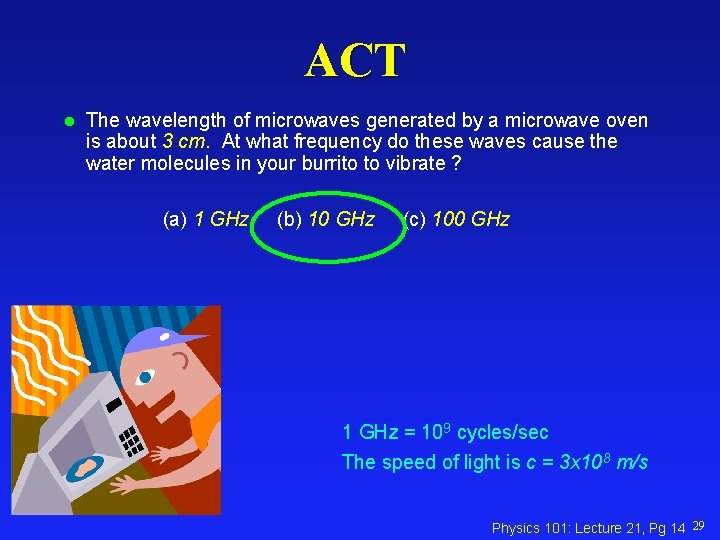ACT l The wavelength of microwaves generated by a microwave oven is about 3 cm. At what frequency do these waves cause the water molecules in your burrito to vibrate ? (a) 1 GHz (b) 10 GHz (c) 100 GHz 1 GHz = 109 cycles/sec The speed of light is c = 3 x 108 m/s Physics 101: Lecture 21, Pg 14 29ACT Solution l Recall that v = f. H H Makes water molecules wiggle O 1 GHz = 109 cycles/sec The speed of light is c = 3 x 108 m/s Physics 101: Lecture 21, Pg 15 30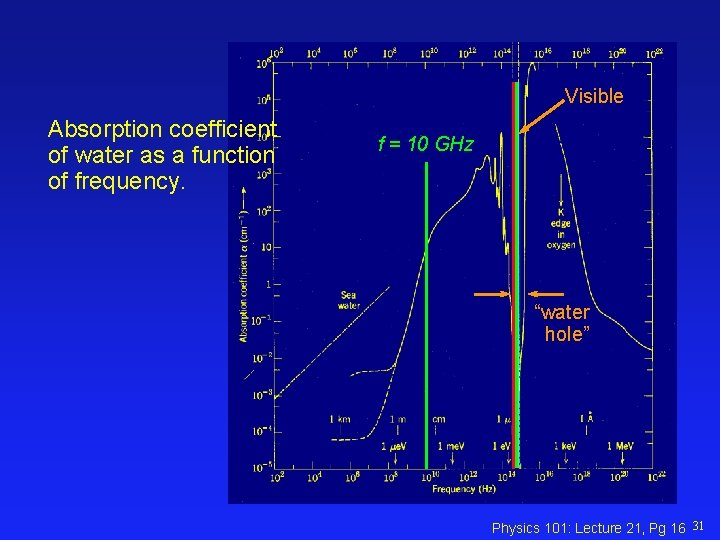Visible Absorption coefficient of water as a function of frequency. f = 10 GHz “water hole” Physics 101: Lecture 21, Pg 16 31Interference and Superposition l When two waves overlap, the amplitudes add. èConstructive: increases amplitude èDestructive: decreases amplitude Physics 101: Lecture 21, Pg 17 34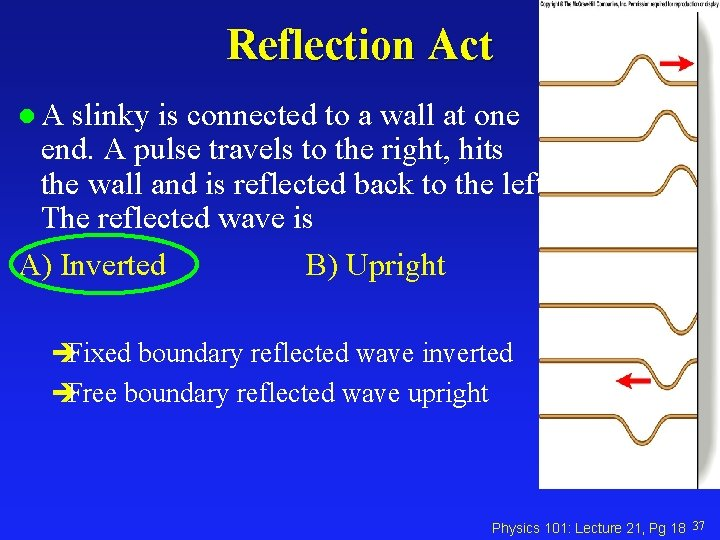Reflection Act l. A slinky is connected to a wall at one end. A pulse travels to the right, hits the wall and is reflected back to the left. The reflected wave is A) Inverted B) Upright èFixed boundary reflected wave inverted èFree boundary reflected wave upright Physics 101: Lecture 21, Pg 18 37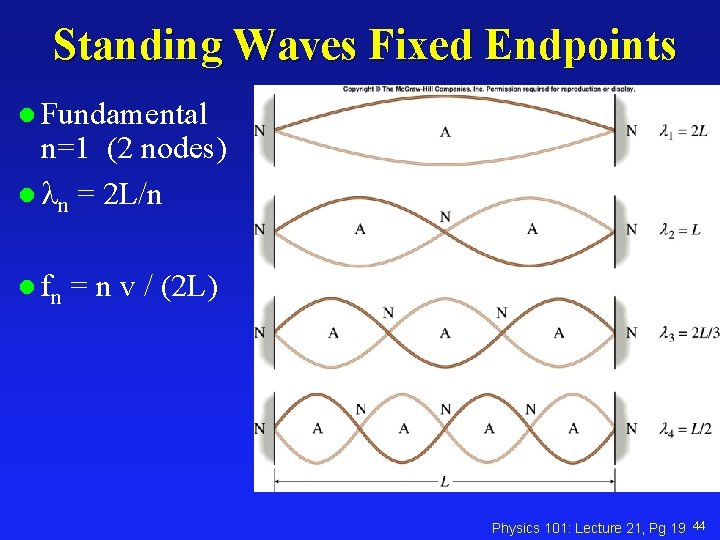Standing Waves Fixed Endpoints l Fundamental n=1 (2 nodes) l n = 2 L/n l fn = n v / (2 L) Physics 101: Lecture 21, Pg 19 44Standing Waves: L = / 2 f 1 = fundamental frequency (lowest possible) A guitar’s E-string has a length of 65 cm and is stretched to a tension of 82 N. If it vibrates with a fundamental frequency of 329. 63 Hz, what is the mass of the string? f = v / tells us v if we know f (frequency) and (wavelength) v= f = 2 (0. 65 m) (329. 63 s-1) = 428. 5 m/s v 2 = T / m m = T / v 2 m= T L / v 2 = 82 (0. 65) / (428. 5)2 = 2. 9 x 10 -4 kg Physics 101: Lecture 21, Pg 20 48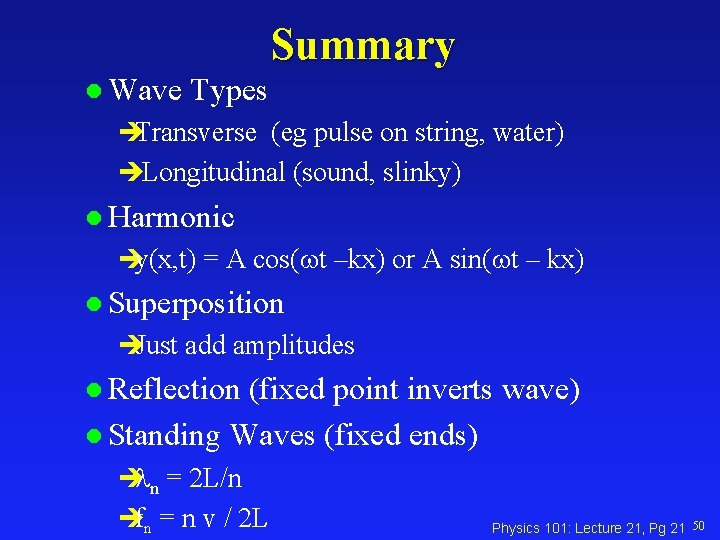Summary l Wave Types èTransverse (eg pulse on string, water) èLongitudinal (sound, slinky) l Harmonic èy(x, t) = A cos(wt –kx) or A sin(wt – kx) l Superposition èJust add amplitudes l Reflection (fixed point inverts wave) l Standing Waves (fixed ends) è n = 2 L/n èfn = n v / 2 L Physics 101: Lecture 21, Pg 21 50